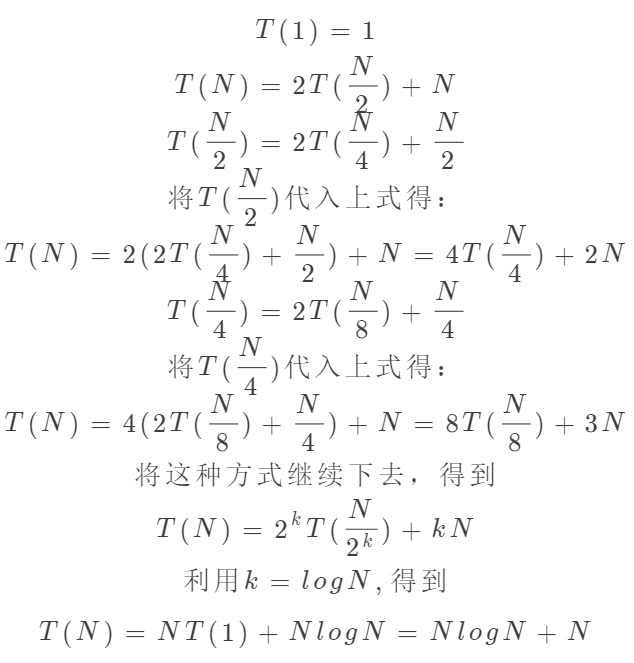# java泛型中使用的排序算法——归并排序及分析

### 二、图解归并排序过程

1. 算法思想：采用分治法:
• 分割：递归地把当前序列平均分割成两半。
• 集成：在保持元素顺序的同时将上一步得到的子序列集成到一起（归并）。
• 归并操作：指的是将两个已经排序的序列合并成一个序列的操作。归并排序算法依赖归并操作。
1. 归并过程：取两个输入数组A、B和一个输出数组C以及3个索引index1,index2,index3分别指向三个数组开始端。并在A[index1]、B[index2]中较小者拷贝到数组C中的下一个位置，相关的索引+1。当A、B中有一个数组走完时，将另一个数组中的元素全部拷贝到数组C中。

### 三、java实现归并排序

public class MergeSort {    public static void main(String[] args) {        Integer[] integers = {7, 1, 13, 9, 15, 5, 8,17};        System.out.println("原序列：" + Arrays.toString(integers));        mergeSort(integers);        System.out.println("排序后：" + Arrays.toString(integers));    }    public static <T extends Comparable<? super T>> void mergeSort(T[] a) {        //因为merge操作是最后一行，所以任何时候只需要一个临时数组        T[] tmpArray = (T[]) new Comparable[a.length];        mergeSort(a, tmpArray, 0, a.length - 1);    }    private static <T extends Comparable<? super T>> void mergeSort(T[] a, T[] tmpArray, int left, int right) {        if (left < right) {            int center = (left + right) / 2;            mergeSort(a, tmpArray, left, center);            mergeSort(a, tmpArray, center + 1, right);            merge(a, tmpArray, left, center + 1, right);        }    }    /**     * 合并左右数据方法     *     * @param a               :原数组     * @param tmpArray        : 临时数组     * @param leftPos         ：左边开始下标     * @param rightPos：右边开始下标     * @param rightEnd：右边结束下标     * @param <T>：元素泛型     */    private static <T extends Comparable<? super T>> void merge(T[] a, T[] tmpArray, int leftPos, int rightPos, int rightEnd) {        int leftEnd = rightPos - 1;        int tmpPos = leftPos;        int numElements = rightEnd - leftPos + 1;        //合并操作        while (leftPos <= leftEnd && rightPos <= rightEnd) {            if (a[leftPos].compareTo(a[rightPos]) <= 0) {                tmpArray[tmpPos++] = a[leftPos++];            } else {                tmpArray[tmpPos++] = a[rightPos++];            }        }        // 复制前半部分        while (leftPos <= leftEnd) {            tmpArray[tmpPos++] = a[leftPos++];        }        //复制后半部分        while (rightPos <= rightEnd) {            tmpArray[tmpPos++] = a[rightPos++];        }        // 回写原数组        for (int i = 0; i < numElements; i++, rightEnd--) {            a[rightEnd] = tmpArray[rightEnd];        }    }}

### 四、归并排序分析1. 时间复杂度：从分析可以看出，归并排序的最好最坏都稳定在O(NlogN)
2. 空间复杂度：需要O(N)个临时空间进行合并操作。
3. 稳定性：稳定。采用分治的思想，每次合并时，在前面的总会先存入临时数组内。

### 六、总结

posted @ 2019-04-29 15:00  9龙  阅读(...)  评论(... 编辑 收藏